S k i l l  i n A R I T H M E T I C Table of Contents | Home | Introduction

Lesson 23

# FRACTIONS INTO DECIMALS

In this Lesson, we will answer the following:

1. What is a "decimal"?
2. If the denominator is not a power of 10, how can we change the fraction to a decimal?
3. Frequent decimals and percents: Half, quarters, eighths, fifths.

Section 2

4. What is a general method for expressing a fraction as decimal? Complete versus incomplete decimals.

 1. What is a "decimal"?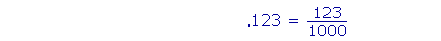A "decimal" is a fraction whose denominator we do not write  but which we understand to be a power of 10. The number of decimal places indicates the number of zeros in the denominator.

The number of decimal places is the number of digits to the right
of the decimal point. (Lesson 3, Question 4.)

Example 1.

 .8 = 8 10 One decimal place; one 0 in the denominator. .08 = 8  100 Two decimal places; two 0's in the denominator. .008 = 8    1000 Three decimal places; three 0's in the denominator. And so on.

The number of decimal places indicates the power of 10.

 Example 2.    Write as a decimal: 614  100,000
 Answer. 614   100,000 = .00614

Five 0's in the denominator indicate five digits after the decimal point.

The five 0's in the denominator is not the number of 0's in the decimal!

Alternatively, in Lesson 10 we introduced the division bar, and in Lesson 3 we saw how to divide a whole number by a power of 10.

 614   100,000 = 614 ÷ 100,000 = .00614

Separate five decimal places.

 Example 3.    Write this mixed number as a decimal:  6 49  100
 Answer.   6 49 100 = 6.49

The whole number 6 does not change. We simply replace the

 common fraction 49 100 with the decimal  .49.

 Example 4.   Write this mixed number with a common fraction:  9.0012
 Answer.   9.0012 = 9 12    10,000

Again, the whole number does not change.  We replace the decimal

 .0012 with the common fraction 12   10,000 .  The decimal  .0012  has four

decimal places.  The denominator  10,000  is a 1 followed by four 0's.

This accounts for fractions whose denominator is already a power of 10.

 2. If the denominator is not a power of 10, how can we change the fraction to a decimal?Make the denominator a power of 10 by multiplying it or dividing it.

 Example 5.   Write 9 25 as a decimal.

Solution.  25 is not a power of 10, but we can easily make it a power of 10 -- 100 -- by multiplying it by 4.  We must also, then, multiply the numerator by 4.Example 6.   Write 45 as a decimal.
 Solution. 45 = 8 10 = .8

We can make 5 into 10 by multiplying it -- and 4 -- by 2.

 Example 7.   Write as a decimal: 7  200
 Answer. 7  200 = 35  1000 = .035

We can make 200 into 1000 by multiplying it -- and 7 -- by 5.

 7  200 = _ 7 _  2 × 100 = 3.5100 , on dividing 7 by 2, = .035, on dividing 3.5 by 100.
 Example 8.   Write as a decimal: 8  200
 Answer. 8  200 = 4 100 = .04

Here, we can change 200 into a power of 10 by dividing it by 2.  We can do this because 8 also is divisible by 2.

Or, again,

 8  200 = _ 8 _  2 × 100 = 4  100 =  .04
 Example 9.   Write as a decimal: 12  400
 Answer. 12  400 = 3 100 = .03

We can change 400 to 100 by dividing it -- and 12 -- by 4.

To summarize:  We go from a larger number to a smaller by dividing (Examples 8 and 9);  from a smaller number to a larger by multiplying (Example 5).

Frequent decimals

The following fractions come up frequently.  The student should know their decimal equivalents.

 12 14 34 18 38 58 78 13 23
 Let us begin with 12 .
 12 = 5 10 = .5  or  .50.
 Next, 14 .  But 14 is half of 12 .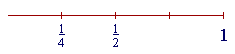Therefore, its decimal will be half of .50 --

 14 =  .25
 And since 34 =  3 × 14 , then
 34 =  3 × .25  =  .75
 Next, 18 .  But 18 is half of 14 .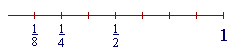Therefore, its decimal will be half of .25  or  .250 --

 18 =  .125

The decimals for the rest of the eighths will be multiples of .125.

Since 3 × 125 = 375,

 38 =  3 × .125  =  .375
 Similarly, 58 will be 5 × 18 =  5 × .125.

5 × 125 = 5 × 100  +  5 × 25 = 500 + 125 = 625.

(Lesson 8)  Therefore,

 58 = .625
 Finally, 78 =  7 × .125.

7 × 125 = 7 × 100  +  7 × 25 = 700 + 175 = 875.

Therefore,

 78 =  .875

These decimals come up frequently.  The student should know how to generate them quickly.

The student should also know the decimals for the fifths:

 15 = 2 10 =  .2

The rest will be the multiples of .2 --

 25 = 2 × 15 = 2 × .2  = .4 35 = 3 × .2  = .6 45 = 4 × .2  = .8
 Example 10.   Write as a decimal:  8 34
 Answer.   8 34 = 8.75

The whole number does not change.  Simply replace the common

 fraction 34 with the decimal .75.

 Example 11.   Write as a decimal: 72

Answer.   First change an improper fraction to a mixed number:

 72 = 3 12 = 3.5

"2 goes into 7 three (3) times (6) with 1 left over."

 Then repalce 12 with .5.

Example 12.    How many times is .25 contained in 3?

 Answer.   .25 = 14 .  And 14 is contained in 1 four times.  (Lesson 19.)
 Therefore, 14 , or .25, will be contained in 3 three times as many times.  It will

be contained 3 × 4 = 12 times.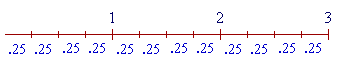Example 13.   How many times is .125 contained in 5?

 Answer.   .125 = 18 .  And 18 is contained in 1 eight times.  Therefore, 18 ,

or .125, will be contained in 5 five times as many times.  It will be contained 5 × 8 = 40 times.

 As for 13 and 23 , they cannot be expressed exactly as a decimal.

However,

 13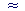0.333

And

 230.667

Frequent percents

From the decimal equivalent of a fraction, we can easily derive the percent:  Move the decimal point two places right(Lesson 3.)  Again, the student should know these.  They come up frequently.

 12 = .50 = 50% 14 = .25 = 25% 34 = .75 = 75% 18 = .125 = 12.5%  (Half of 14 .) 38 = .375 = 37.5% 58 = .625 = 62.5% 78 = .875 = 87.5% 15 = .2 = 20% 25 = .4 = 40% 35 = .6 = 60% 45 = .8 = 80%

In addition, the student should know

 13 =  33 13 % 23 =  66 23 %

At this point, please "turn" the page and do some Problems.

or

Continue on to the next Section.

www.proyectosalonhogar.com